# Date and Time in Excel

Contents

### How are date and time stored and formatted?

No matter how date and/or time are formatted and possibly edited, the underlying values saved in cells are still decimal numbers.

Given that Excel’s era starts on January 1, 1900, if we enter number 1 in the cell and format it as a date, the date we will see is in fact January 1, 1900.Zero will provide for a nonexistent date of January 0, 1900, and a negative number will result in errors when formatted as a date: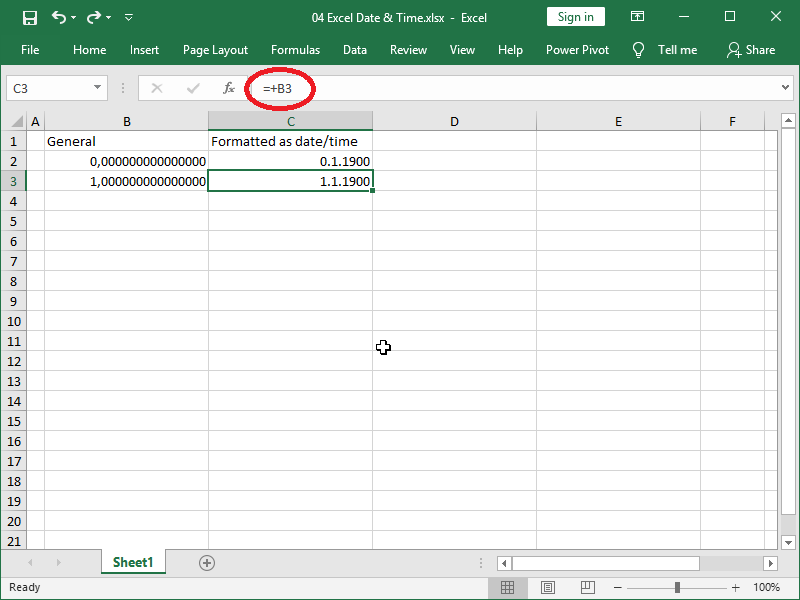Knowing that, we can use basic mathematical operations on those numbers:

• January 1, 1900 (1) + 30 equals January 31, 1900 (31)
• January 1, 1900 (1) multiplied by 365 days and 120 years and corrected for leap years equals January 1, 2020 (43831)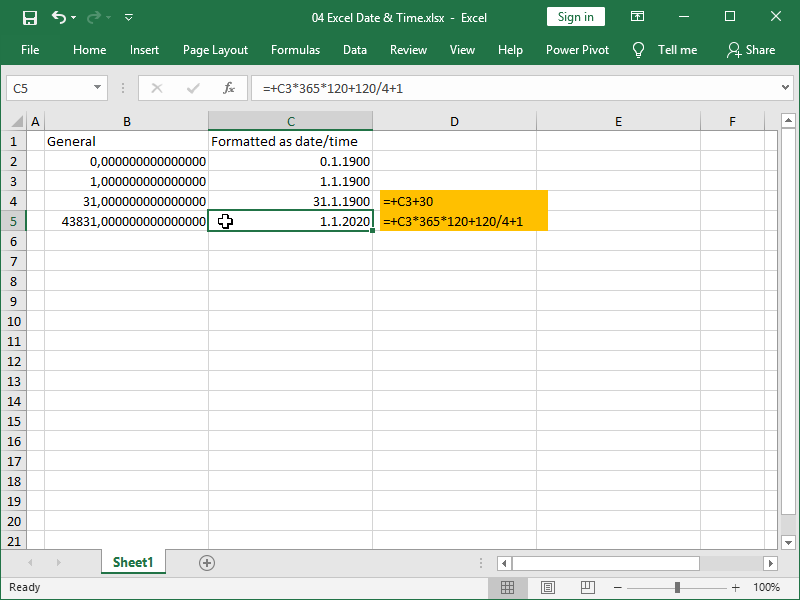Hours, minutes, and seconds work much the same way; they are in fact decimal points: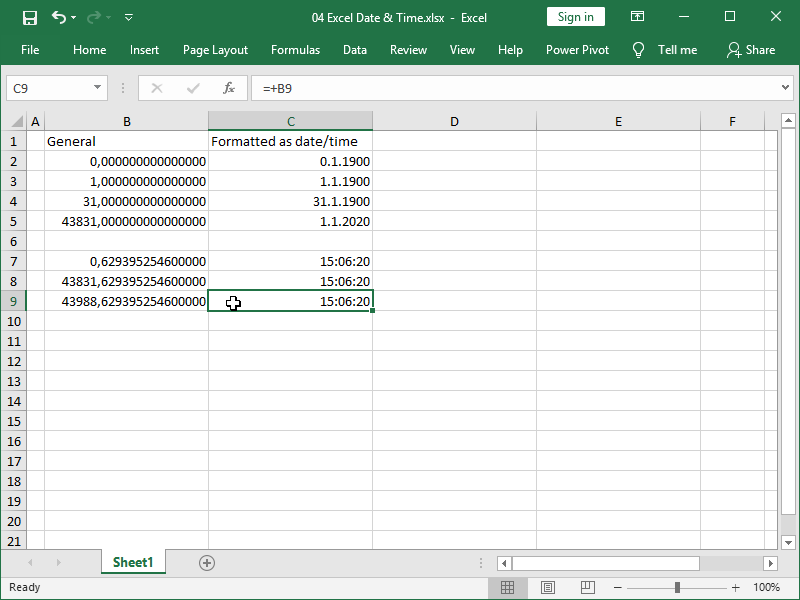Note that whatever the date (no date, January 1, 2020, or June 6, 2020), the cell formatted as time will show the same figure. In fact, you will often find the time of day saved as shown in cell B7.

Here is how those numbers look if we format them as both date and time: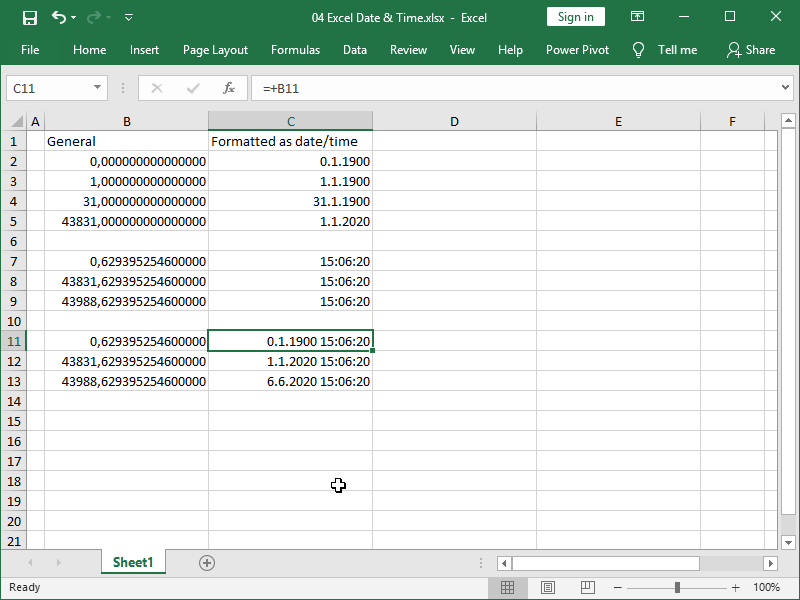Both date and time notations here are my local notations. However, it’s rather trivial to switch between the notations of different countries in Excel. After we select some data, we can right-click and choose the Format Cells option in the menu. In the pop-up, under Date, any Locale (location) available in Excel can be chosen, and then one of the available formats for that locale under Type: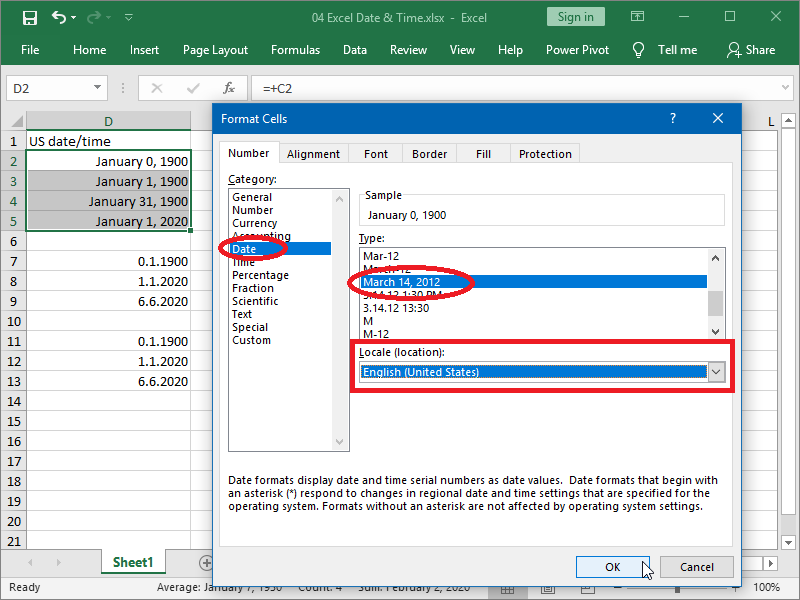It works much the same way for Time: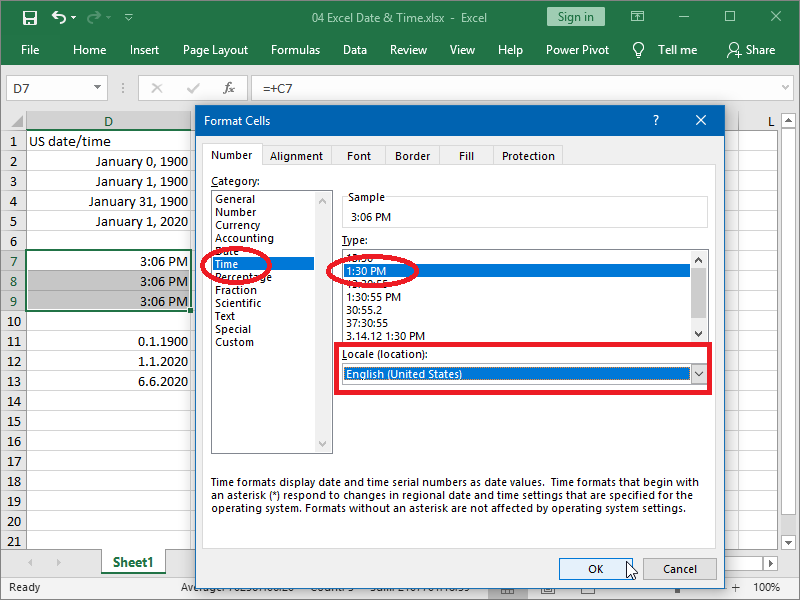In addition to the preset options available for formatting dates in Excel, for specific use cases, additional custom date formats can also be created (coded) or existing ones modified: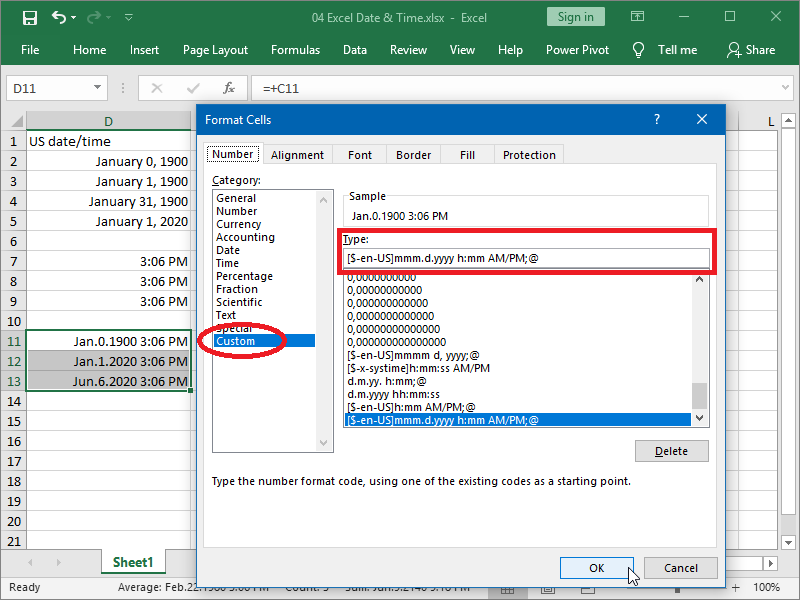All of this doesn’t mean you will be entering dates, hours, and minutes in cells in the shown decimal format. Excel, in fact, goes above and beyond trying to recognize any date or time you’ve tried to input, and if you do so in any standard format for your locale, it will save the date or time value you’ve tried to type in while at the same time formatting the cell as a date and/or time.

For example, if I enter the text “06.06.2020” in the cell E15, Excel will assume I’m trying to enter a date, save the value 43988, and format it as date. If I try to enter that cell again, I will be editing figure “6.6.2020”. If I don’t do something unexpected, I may never find out that I am actually dealing with number 43988.

The same thing will happen if I try to enter time: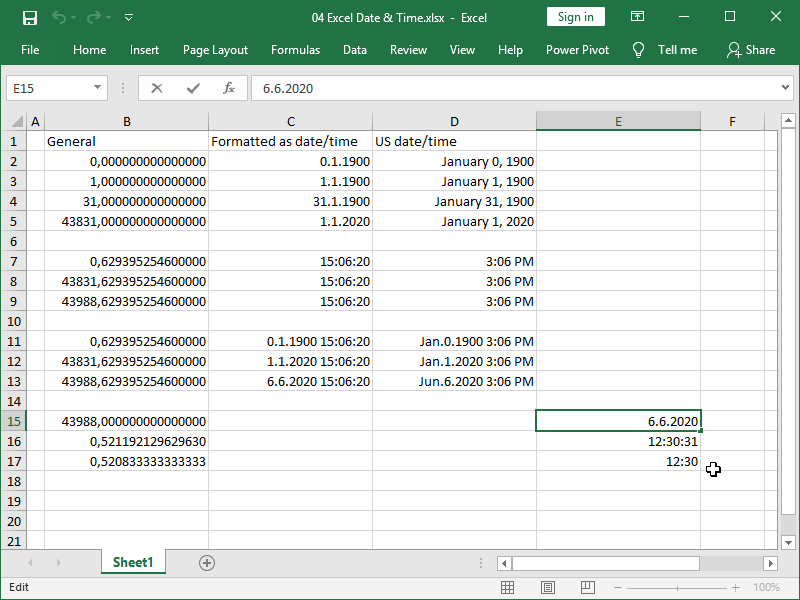### How to convert text to date and time?

In Excel, dates and times formatted as text are often encountered. If we want to make use of that data, we will first have to convert it into values.

The DATEVALUE function converts a date that is stored as text to a serial number that Excel recognizes as a date.

The TIMEVALUE function converts a time that is stored as text to a serial number that Excel recognizes as a time.

Here is how we can apply those functions: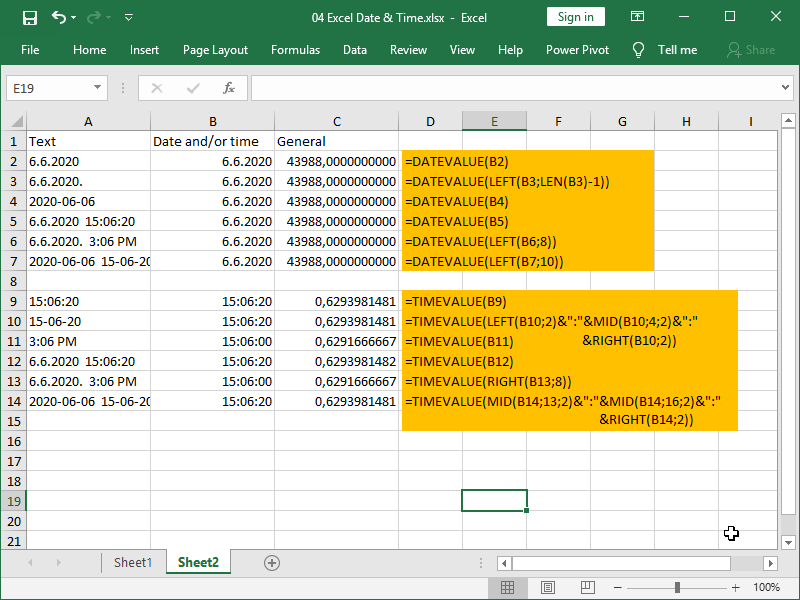When date and time are stored as text but still formatted in the way dates are usually formatted in your Excel locale, Excel will have little issue converting them.

The further we move from formats that Excel will recognize, the more complications arise. I.e., an increasing number of mostly text functions will have to be used in order to convert that text into something Excel will recognize as a date and/or time.

For example, I will not be able to convert the text “Jun.6.2020” to a number, as Jun as a format of month is not present in my locale. In order to accomplish that, I would have to:

Another thing to note in the example above is the time returned to cells B11 and B13. Here, part of the information was lost with prior conversion to text and can’t be retrieved anymore; text 3:06 PM doesn’t contain seconds information any more.

If we want to return both date and time values into the same cell, we can add up the DATEVALUE and the TIMEVALUE returns: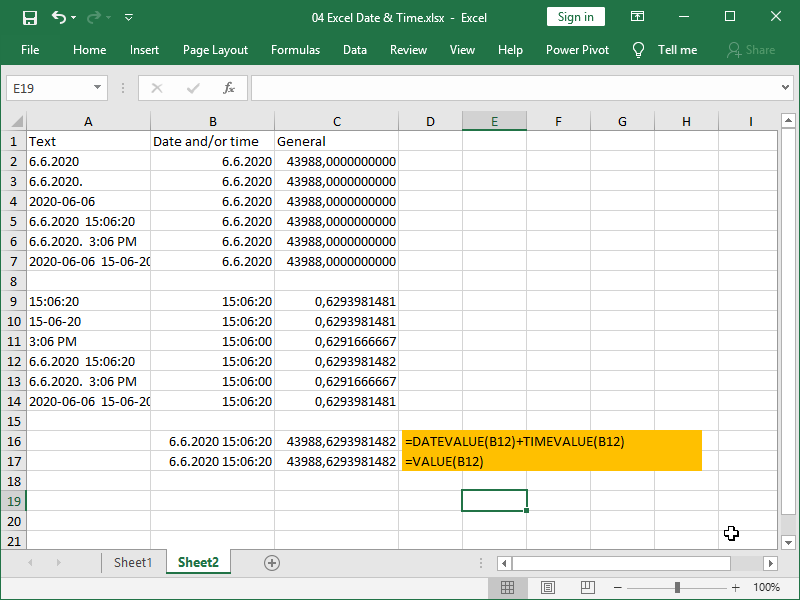As shown by the formula in cell B17, in principle we can also use the VALUE function for such tasks, but note that using the VALUE function is sometimes easier to get false readings.

### Basic date and time functions

You will also encounter years, months, days, and even hours, minutes, and seconds formatted and saved as integer numbers. This format is sometimes used to calculate differences between years, months, days, etc.: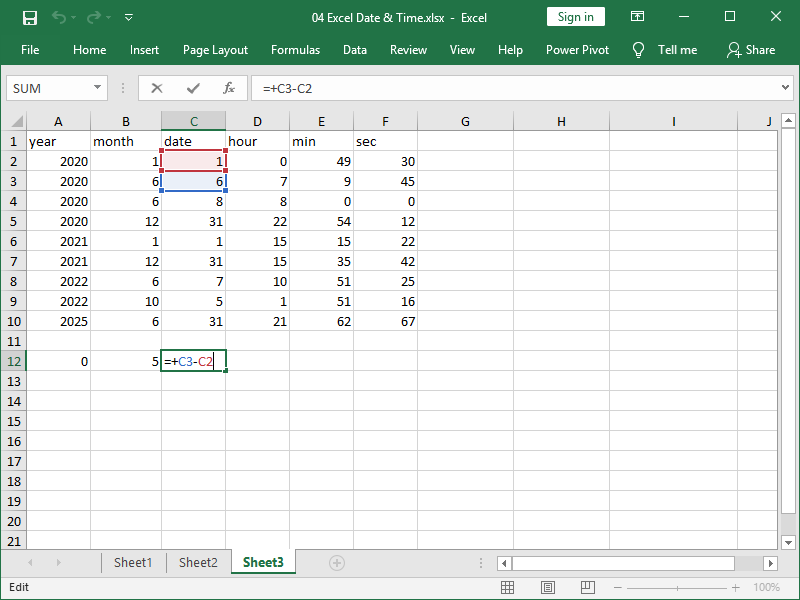The DATE function can be used to convert those integer numbers to dates in Excel format.

The TIME function can be used to convert those integer numbers to time in Excel format.

Note that both functions will correctly interpret higher than expected values, i.e., 62 minutes will be interpreted as 1 hour and 2 minutes. However, 25 hours will be interpreted as one hour.

The DATE and TIME functions can also be combined: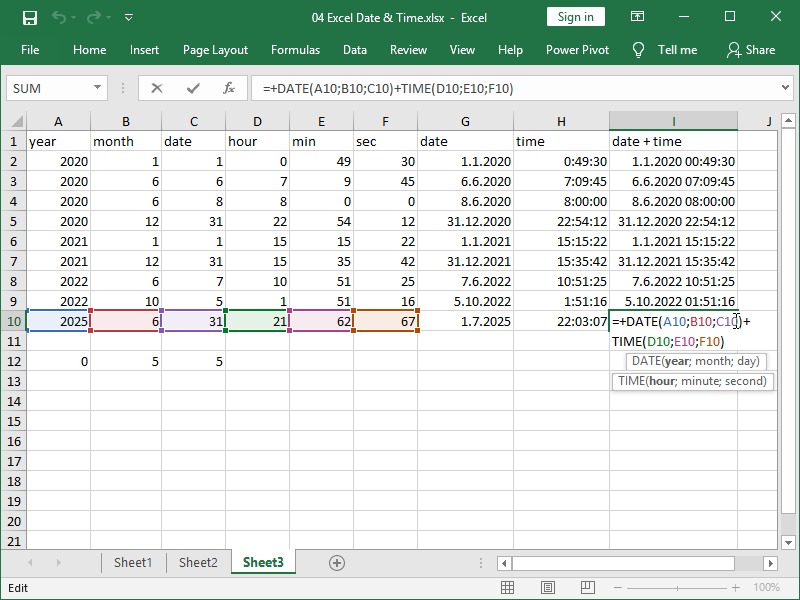If we want to do the opposite, i.e., if we want to extract date and time components as integer numbers, we will need to use the YEAR, MONTH, DAY, HOUR, MINUTE, and SECOND functions: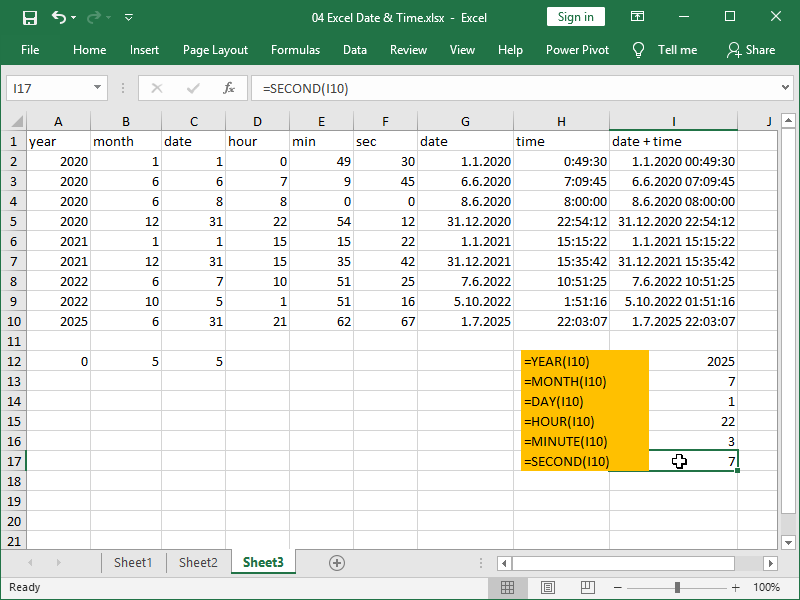The EOMONTH function returns the last day of the month in relation to the start date. Check out examples and applications in this article, and learn how to generate a sequence of month-end dates in this article.

The WEEKNUM function will return an integer number representing the week of the year, and the ISOWEEKNUM function will do the same according to the ISO 8601 standard, without fractional weeks, with =WEEKNUM(date;21) being equivalent to ISOWEEKNUM (date).

The WEEKDAY function will return an integer number representing the day of the week.

The DAYS function will return an integer number of days between two dates.

The DAYS360 function will return an integer number of days between two dates based on a 360-day year.

The YEARFRAC function will return a decimal number: the number of days between two dates divided by the number of days in the year.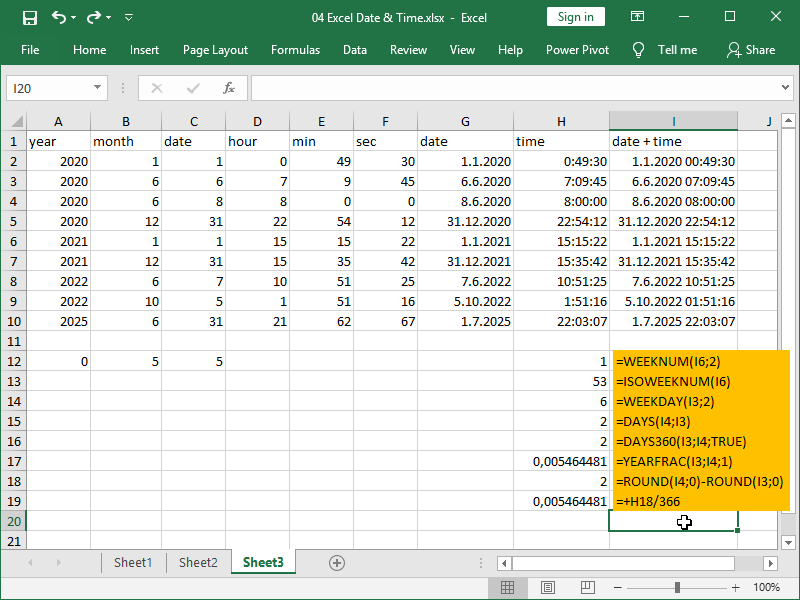Be careful while using the DAYS, DAYS360, and YEARFRAC functions; those are not basic functions anymore. We are now in the territory of interest calculation, and while those functions can be used for general purposes, be sure of what you are set up to do and what they are actually doing. If unsure, you can return the number of days between two days using basic arithmetic, such as shown in cells H18 and H19.

Be careful while using the WEEKNUM, WEEKDAY, and DAYS360 functions; the default returns are based on the US calendar and possibly not what you need!

For example, the formula =WEEKDAY(I3) gives us the default return, the integer number 7, as Saturday is the 7th day of the US week. Formula =WEEKDAY(I3;1) returns the integer number 7, given that we are now, with return type specified as 1, asking for our weeks to start on Sunday. Only the formula =WEEKDAY(I3;2) will return the integer number 6, given that we are now, with return type specified as 2, asking for our weeks to start on Monday.

With this kind of function, make use of pop-ups with available options and their descriptions: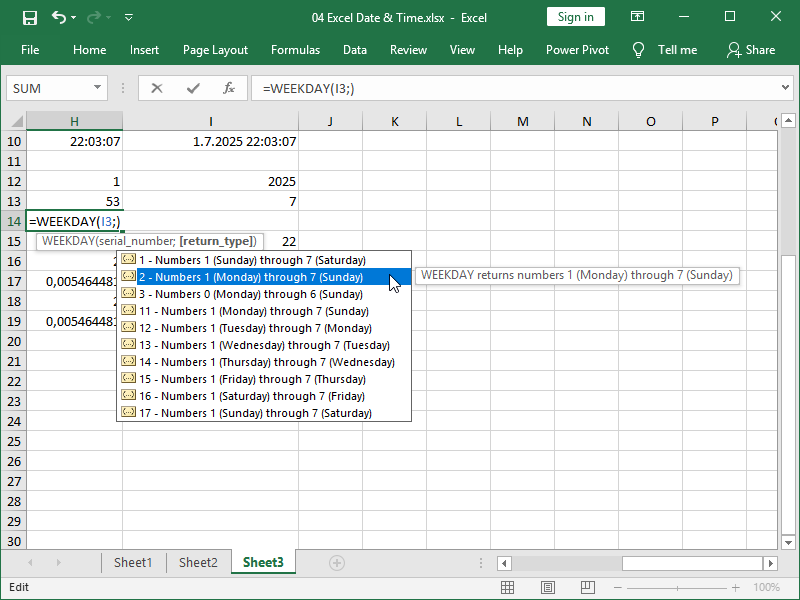If we want to read system time, we can use the TODAY and NOW functions.

The TODAY function returns the current date.

The NOW function returns the current date and time.

You will not be inserting arguments between (); those functions will read system time. One example of what we can do with those is that we can return them into the TEXT function, format that text as date and time, and create a “Printed on” cell: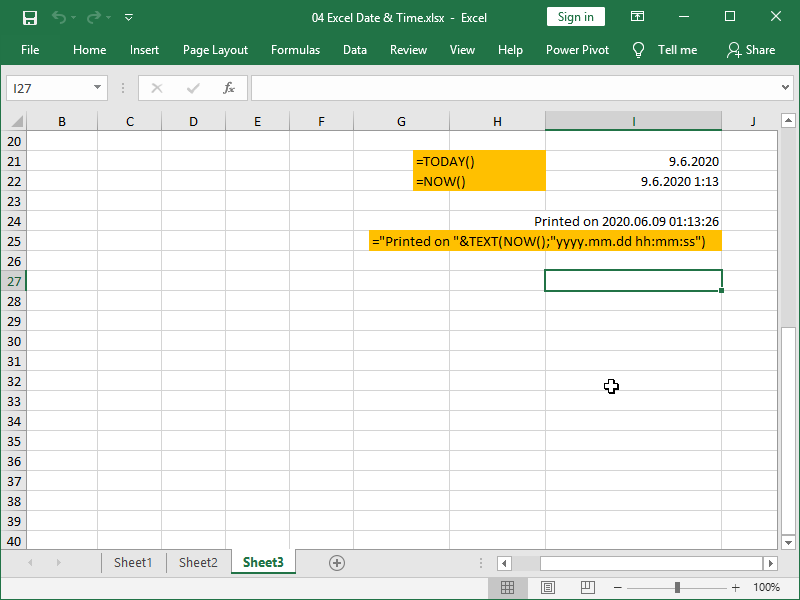Be careful while using the TODAY and NOW functions; those will recalculate often.